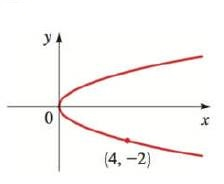Chapter 12.1, Problem 55E### Algebra and Trigonometry (MindTap ...

4th Edition
James Stewart + 2 others
ISBN: 9781305071742

#### Solutions

Chapter
Section### Algebra and Trigonometry (MindTap ...

4th Edition
James Stewart + 2 others
ISBN: 9781305071742
Textbook Problem

# 49-58 ■ Finding the Equation of a Parabola Find an equation of the parabola whose graph is shown.To determine

To find:

The equation for the parabola of given figure.

Explanation

Given:

The equation of the parabola whose graph is shown below

Approach:

The general equation for a parabola with a horizontal axis having the vertex is

y2=4px …… (1)

Here, p is the distance from the origin and the vertex is V(0,0).

The focus is F(p,0) and the directrix line is x=p

The parabola opens to the upward if p>0 or to the downward if p<0.

Calculation:

From the given figure,

The vertex is V(0,0) and the point (4,2) lies on the parabola.

Substitute 4 for x and 2 for y in equation (1)

### Still sussing out bartleby?

Check out a sample textbook solution.

See a sample solution

#### The Solution to Your Study Problems

Bartleby provides explanations to thousands of textbook problems written by our experts, many with advanced degrees!

Get Started